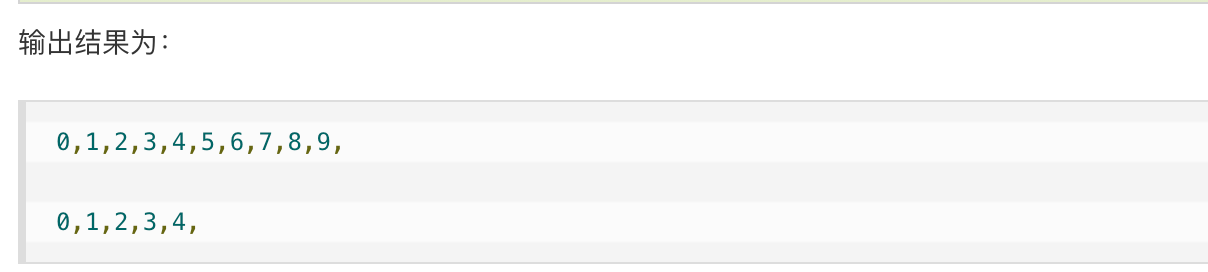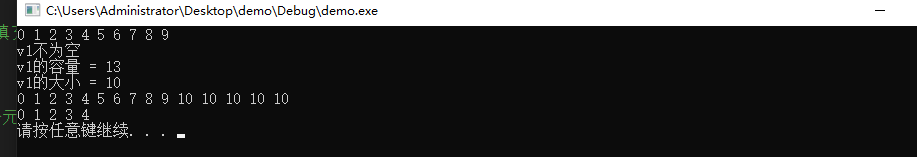• C++vector自定义大小

千次阅读 2020-03-29 10:46:17
如何在定义Vector的时候指定大小
1. vector指定大小（前者必须是定义vector时）
vector<int>vc(5);//size为5，值默认为0
cout<<vc.size()<<endl;
for(int i=0; i<vc.size(); i++)
cout<<vc[i];

vector<int>vc;
vc.resize(5); //size为5，值默认为0

1. 指定大小并赋相同初值（前者必须是定义vector时）
vector<int>vc(5,9); //size为5，初值为9
cout<<vc.size()<<endl;
for(int i=0; i<vc.size(); i++)
cout<<vc[i];

vector<int>vc;
vc.resize(5,9);

1. 二维vector指定大小
vector<vector<int> >vc(5);//指定行数
for(int i=0; i<vc.size(); i++)
vc[i].resize(i);	//指定列数(每行列数可以不同)
//vc[i].resize(i,9);//指定列数并对该行赋相同初值

vector<vector<bool> >inq;
vector<bool> tmp(m, false);
inq.resize(n, tmp);//nxm，值全为false

vector<vector >inq(n, vector(5));

注意：指定大小后，范围内的元素要么默认是0，要么指定元素，此时紧接着push_back将在vc[size]处尾插。
注意：上述代码使用resize仅是用来初始化，并未介绍详细用法。
https://blog.csdn.net/Gease_Gg/article/details/83785584?utm_source=app

展开全文• C++ 获取std::vector 长度 大小

千次阅读 2021-10-08 21:46:44
vector> #include <iostream> using namespace std; int main() { vector<int>obj;//创建一个向量存储容器 int for(int i=0;i<10;i++) // push_back(elem)在数组最后添加数据 { obj.push.....

obj.size()

#include <string.h>
#include <vector>
#include <iostream>
using namespace std;

int main()
{
vector<int>obj;//创建一个向量存储容器 int
for(int i=0;i<10;i++) // push_back(elem)在数组最后添加数据
{
obj.push_back(i);
cout<<obj[i]<<",";
}

for(int i=0;i<5;i++)//去掉数组最后一个数据
{
obj.pop_back();
}

cout<<"\n"<<endl;

for(int i=0;i<obj.size();i++)//size()容器中实际数据个数
{
cout<<obj[i]<<",";
}

return 0;
}展开全文c++
• vector容量和大小

千次阅读 2019-07-03 17:50:09
vector容量和大小 功能描述： 对vector容器的容量和大小操作 函数原型： empty(); //判断容器是否为空 capacity(); //容器的容量 size(); //返回容器中元素的个数 resize(int...

vector容量和大小

功能描述：

• 对vector容器的容量和大小操作

函数原型：

• empty(); //判断容器是否为空

• capacity(); //容器的容量

• size(); //返回容器中元素的个数

• resize(int num); //重新指定容器的长度为num，若容器变长，则以默认值填充新位置。

​ //如果容器变短，则末尾超出容器长度的元素被删除。

• resize(int num, elem); //重新指定容器的长度为num，若容器变长，则以elem值填充新位置。

​ //如果容器变短，则末尾超出容器长度的元素被删除

测试代码

#include <iostream>
using namespace std;
#include <string>

#include <vector>

void printVector(vector<int>& v) {

for (vector<int>::iterator it = v.begin(); it != v.end(); it++) {
cout << *it << " ";
}
cout << endl;
}

void test01()
{
vector<int> v1;
for (int i = 0; i < 10; i++)
{
v1.push_back(i);
}
printVector(v1);

if (v1.empty())
{
cout << "v1为空" << endl;
}
else
{
cout << "v1不为空" << endl;
cout << "v1的容量 = " << v1.capacity() << endl;
cout << "v1的大小 = " << v1.size() << endl;
}

//resize 重新指定大小 ，若指定的更大，默认用0填充新位置，可以利用重载版本替换默认填充
v1.resize(15, 10);
printVector(v1);

//resize 重新指定大小 ，若指定的更小，超出部分元素被删除
v1.resize(5);
printVector(v1);
}

int main() {

test01();

system("pause");

return 0;
}

测试结果总结

• 判断是否为空 — empty
• 返回元素个数 — size
• 返回容器容量 — capacity
• 重新指定大小 — resize
展开全文vector 容器 C++ STL capacity
• #include&...vector&gt; using namespace std; int main(){ vector&lt;int&gt; a; cout &lt;&lt; a.size() &lt;&lt; endl;//0 a.push_back(0); a.push_back(1)...
#include<iostream>
#include<string>
#include<vector>
using namespace std;
int main(){
vector<int> a;
cout << a.size() << endl;//0
a.push_back(0);
a.push_back(1);
a.push_back(2);
a.resize(2);
cout << a.size() << endl;//2
cout << a << a << endl;//0 1 输出a则报错
return 0;
}

展开全文• 在对二维vectorvector&lt; &lt;vector&lt;int&gt;&gt; vec用push_back存放数据时，如vec[i].push_back()会出现下标越界。这是因为此时的vec为空，无论是vec还是vec[i]的size都是0，这个时候...STL 二维vector
• C++二维vector初始化大小方法

千次阅读 2020-07-09 14:20:28
1.初始化二维vector，为r*c的vector，所有值为0. ...2.用resize()来控制大小 vector<vector<int> > res; res.resize(r);//r行 for (int k = 0; k < r; ++k){ res[k].resize(c);//每行为c列 } ...c++
• 前言 vector是一个动态的数组，我们最常使用push_back()向vector中添加元素。...如何初始化vector到我们想要的大小呢。这就需要用到vector的resizeh函数了。 resize 首先看resize的函数原型 void ...C++ vector
• C++vector容器vector容量和大小

千次阅读 2020-09-04 15:15:15
C++vector容器vector容量和大小 功能描述： 对vector容器的容量和大小操作 函数原型： empty(); //判断容器是否为空 capacity(); //容器的容量 size(); //返回容器中元素的个数 resize(int num); //重新...c++
• vector<int> obj(size); 或 vector<int> obj; obj.resize(size); 2. 二维 vector<vector<int> > obj(rsize, vector<int>(lsize, 0)); 或 vector<vector<int>> ob...
• 关于vector大小（size）和容量（capacity）总结

万次阅读 多人点赞 2016-04-08 22:37:03
主要介绍了vector 容器大小相关的操作函数（size、max_szie、capacity），同时介绍了容器大小超出容器capacity能力会内存重新分配以及iterator失效简单分析。同时出了避免内存重新分配的两种方法。size capacity max_size vector
• （1）int的大小是4，定义vector<int>vec,vec中有一个元素，sizeof(vec)=20,如果有1000个元素，则sizeof(vec)是多少？ #include <iostream> #include <vector> using namespace std; int main() ...
• 做了好多测试，发现在使用reserve手动给vector容器分配大小后，原来容器的迭代器，引用全部都没有失效 这我就不明白了，在使用insert时，导致内存重新分配时，迭代器会失效，而是用reserve却没有。 源码看的一脸懵...c++ vector容器 迭代器
• 函数capacity返回vector的当前容量。 函数size返回vector的实际包含的元素的个数。 // by鸟哥 qq1833183060 #include <iostream> #include <vector> int main() { std::vector<int> v{3,5}; v....
• ** 一、定义一个一维vector数组v1，数组大小为n，初始值为0** 初试化数组长度 const int m = 100; vector<int> v1(m); //size = 100 初始化数组大小和值 vector<int> v1(m, 0); //size = 100; ...
• Vector公司标定工具CANape介绍，包括基本标定原理、CANape主要功能、使用方法、应用案例、优势等
• 名字为vec，大小为n*m，初始值为0的二维vectorvector<vector<int> > vec(n, vector<int>(m, 0)); 2.用resize()来控制大小 vector<vector<int> > vec; vec.resize(n);//n...
• ！ 对于二维vector变量，vv.size()的值vector的行数，vv[i].size()的值是vector的列数！！ 一个二维向量每个元素的长度可以不同，例如 [cpp] view plain co
• 标准库类型vector表示某种类型对象的集合，集合中每个对象都有一个与之对应的索引，用以访问对象。要想使用vector，必须包含头文件vector，并且需要声明using std::vector。/*--------------------创建和初始化...C++
• string： string str("hello world"); str.size(); str.length(); strlen(str.c_str()); end(str) - begin(str) 固定容器大小的array： sizeof(array)/sizeof(array);...vector: ...vector是可变大小容...
• 由书上的知识可知，C++中的二维数组实质上是一维数组里存储的元素仍然是一维数组，而且在定义的时候必须指定数组的大小，很显然无法满足我们的需要。 经过查找网上的资料，发现还有一种方法是利用new运算符建立动态...opencv Saliency
• 问：“vector大小是如何增长的？” 答：“自动增长的” 问：“增长规律是？” 答： 。。。。。。那么， 今天就来探究一下vector容器的大小增长规律：vector中得到大小的的函数有以下几个方法作用size()返回容器的...size
• C++ STL中提供的容器是利用模板实现的，可以很方便的重载各种类型，当然也可以在容器中嵌套容器（但是这种嵌套的方法运行通常效率不高），vector容器中嵌套vector容器可以在初始化时事先声明大小，申请足够大的内存...c++
• C++——二维vector初始化大小方法

万次阅读 多人点赞 2017-05-01 11:24:35
初始化二维vector，为r*c的vector，所有值为0.1.直接用初始化方法（刚开始没想到） vector<vector<int>> newOne(r, ...2.用resize()来控制大小 vector<vector<int> > res; res.resize(r);//r行 for (int k = 0; k ;C++ 二维vector
• 在一个二维数组中，每一行都按照从左到右递增的顺序排序，每一列都按照从上到下递增的顺序排序。... bool Find(int target, vector&lt;vector&lt;int&gt; &gt; array) {bool res=false; ...
• vector

千次阅读 多人点赞 2019-06-24 19:49:29
vector(向量): C++中的一种数据结构,确切的说是一个类.它相当于一个动态的数组,当程序员无法知道自己需要的数组的规模多大时,用其来解决问题可以达到最大节约空间的目的. 前提： #include<vector> using ...vector STL之vector vector详解
• vector(向量): C++中的一种数据结构,确切的说是一个类.它相当于一个动态的数组,当程序员无法知道自己需要的数组的规模多大时,用其来解决问题可以达到最大节约空间的目的.头文件：加上 #include&lt;vector&...动态数组存储
• vector增删数据容器大小变化

千次阅读 2014-11-16 17:27:01
vector容器增删改查数据时容量大小的变化，当增加元素时若容器不够大则将容器扩展为原来大小的1.5倍；删除元素时容器大小不发生任何变化。测试 stl vector容器...